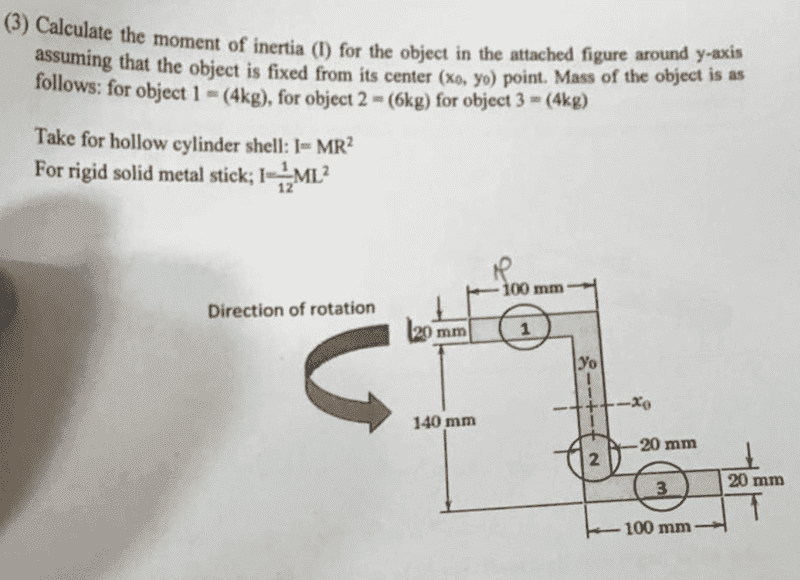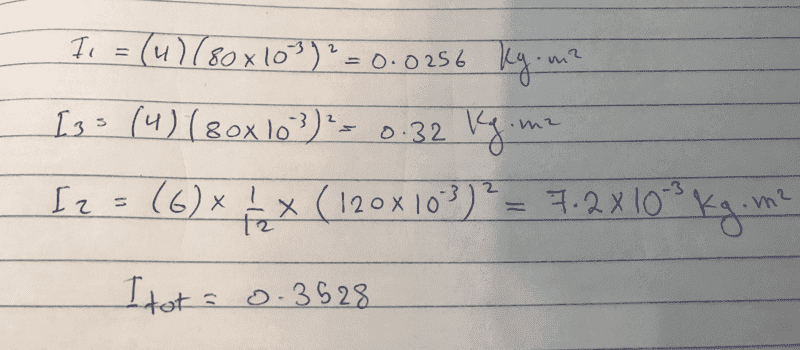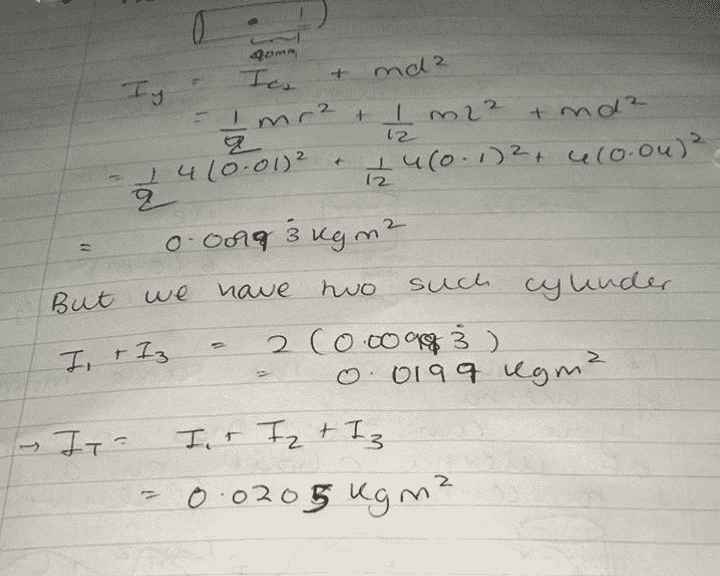# Rotational inertia hoop around a central axis

SakuRERE

## Homework Statement## Homework Equations

I= mr^2 for a hoop around a central axis
I= 1/12 (m)(l)^2 for thin rod about axis through center perpendicular to lenght

## The Attempt at a Solution

I am totally confused. i said first that the three masses each will make a hoop shape so i found I=mr^2 for each mass and regarding the rod i can't imagine any thing!

#### Attachments

Homework Helper
Gold Member
2022 Award
the three masses each will make a hoop shape
For the purpose of moment of inertia, the shape is that of the stationary object, not the volume it will describe in space as it rotates.
It is unclear from the question, but I would guess this is supposed to be a lamina. The MoI of a rectangular lamina about a central axis parallel to one pair of sides is the same as for a stick. The width parallel to the axis is not relevant.

SakuRERE
For the purpose of moment of inertia, the shape is that of the stationary object, not the volume it will describe in space as it rotates.
It is unclear from the question, but I would guess this is supposed to be a lamina. The MoI of a rectangular lamina about a central axis parallel to one pair of sides is the same as for a stick. The width parallel to the axis is not relevant.
central axis parallel to one pair of sides is the same as for a stick
do you mean both have same formula? if yes what is it?

Homework Helper
Gold Member
2022 Award
do you mean both have same formula? if yes what is it?
It's the formula you quoted for a stick, taking the length at right angles to the axis.

SakuRERE
It's the formula you quoted for a stick, taking the length at right angles to the axis.

what about the mass of the rod, what will be the value I am going to substitute in the 1/12(m)(l)^2 ?
and am I going to separate the rods into three segments?

Homework Helper
Gold Member
2022 Award
am I going to separate the rods into three segments?
Yes. Cut the lamina into three rectangles. There is more than one way. Pick the most convenient.
The mass in each case is the mass of the rectangle.

SakuRERE
okay, but what i don't get is what is the mass of the rectangular. now imagine i am working on the upper segment. the mass would it be 4kg?? i don't know really if the 4 kg is the mass of the whole rectangular or only the mass of the part of the circle which is included in the segment itself! if it was for all the rectangular then i think it's somehow easier than the second assuming. because on the second assumption the mass is not distributed equally through the rectangular, and i have never face such a problem like this ( my knowledge of rotational inertia is really basic you can say we are studying a high school level only without going through integrals and stuff).
I am interested to know what's level of difficulty do you see in this question?

SakuRERE
also, would you mind suggesting to me any useful videos on youtube or whatever about similar problems? or even a book including such problems, because i searched for somehow same ideas but couldn't find, it would be nice from you sir to help with it

Homework Helper
Gold Member
2022 Award
what i don't get is what is the mass of the rectangular.
Hmm.. I see what you mean. It is unclear what is included in each object. No matter which way you cut it, the densities vary.
The way the dimensions are given, it looks like the square where 1 and 2 meet belongs to 1, but where 2 and 3 meet it seems to be included in both.

•SakuRERE
SakuRERE
The way the dimensions are given, it looks like the square where 1 and 2 meet belongs to 1, but where 2 and 3 meet it seems to be included in both.
would you mind explain it more, i don't get which common squares you are refereing to?

Homework Helper
Gold Member
2022 Award
would you mind explain it more, i don't get which common squares you are refereing to?
Objects 1 and 2 meet at a 20mm x 20mm square. If all parts of the lamina were of the same density you could include this in object 1 (making it 100 x 20) or in object 2 (making object 1 80 x 20). Either way should give the same total. But since (it seems) the objects have different densities, the choice makes a difference to the total MoI.
Because the vertical dimension for object 2 (140mm) is given in a way that excludes that 20x20 square, it is reasonable to take that square as being part of object 1. But at the joint of objects 2 and 3 it is ambiguous. The 140mm suggests this square is part of object 2, but the 100mm length for object 3 implies it is part of object 3.

•SakuRERE
Homework Helper
Gold Member
2022 Award
only the mass of the part of the circle
I did not understand this comment before. I just realized you mean the circles around the object numbers. I am fairly sure these are just to show that the numbers are labels. There are no circular objects.
If the circles were objects then you would need to know exactly where they are, and we are not given their positions.

•SakuRERE
SakuRERE
I did not understand this comment before. I just realized you mean the circles around the object numbers. I am fairly sure these are just to show that the numbers are labels. There are no circular objects.
If the circles were objects then you would need to know exactly where they are, and we are not given their positions.
i thought that these are circles or (discs shape) because our professor said he will be providing us with necessary laws of MoI for each shape found in the diagram. so i said there is nothing other than the circles to make the hollow cylinder shell shape so that we use (I=MR^2). or is it that the upper segment of the rod and the lower segment will make that shape ( i starting to see it from another view)

Homework Helper
Gold Member
2022 Award
there is nothing other than the circles to make the hollow cylinder shell shape so that we use (I=MR^2).
There are many snags in interpreting the circles as being the objects.
- We are not given their exact positions.
- We are not given their radii
- If they are cylinders, with the given axis of rotation we would need to know their length (and a more complicated formula would apply)
- Even if they are just rings, the mr2 formula is for rotation about an axis normal to the plane of the ring (or cylinder). The axis here is in the plane of the rings.
So I cannot see the relevance of that particular formula. Did the prof imply that all provided formulae would be needed?

SakuRERE
There are many snags in interpreting the circles as being the objects.
- We are not given their exact positions.
- We are not given their radii
- If they are cylinders, with the given axis of rotation we would need to know their length (and a more complicated formula would apply)
- Even if they are just rings, the mr2 formula is for rotation about an axis normal to the plane of the ring (or cylinder). The axis here is in the plane of the rings.
So I cannot see the relevance of that particular formula. Did the prof imply that all provided formulae would be needed?could this be right by any chance?

#### Attachments

Homework Helper
Gold Member
2022 Award
could this be right by any chance?
No.
There is nothing special about the longer of the two dimensions of a rectangle. What matters for the formula is which dimension is normal to the axis of rotation. The length parallel to the axis does not feature in the formula.

SakuRERE
w
No.
There is nothing special about the longer of the two dimensions of a rectangle. What matters for the formula is which dimension is normal to the axis of rotation. The length parallel to the axis does not feature in the formula.